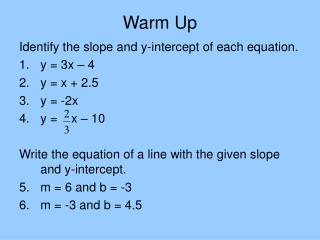DownloadDownload PresentationWarm Up

# Warm Up

Download Presentation## Warm Up

- - - - - - - - - - - - - - - - - - - - - - - - - - - E N D - - - - - - - - - - - - - - - - - - - - - - - - - - -
##### Presentation Transcript

1. Warm Up Identify the slope and y-intercept of each equation. • y = 3x – 4 • y = x + 2.5 • y = -2x • y = x – 10 Write the equation of a line with the given slope and y-intercept. • m = 6 and b = -3 • m = -3 and b = 4.5

2. Writing Equations in Slope-Intercept Form Review. Solve each equation for x. • 3x – 5 = 7 • 4 – x = 15 • -2x + 3 = -9 We are going to use a similar process to write the equations of lines in slope-intercept form.

3. Ex1 Identify the slope and y-intercept in the equation y = 3x – 4. Suppose you had an equation written like this: 3x + 2y = 8. You can’t identify the slope and intercept as easily, so you need to re-write the equation in y = mx + b form.

4. Step 1: Get y’s together on the left side and everything else on the right. Do this by adding the opposite to the x terms. 3x + 2y = 8 -3x -3x 2y = 8 – 3x Step 2: Rearrange the terms so that the equation is starting to resemble y = mx + b 2y = -3x + 8(be sure to watch signs!) Step 3: Divide everything by the coefficient of the y-term and simplify. 2y = -3x + 8 222 y = x + 4

5. Ex2Write in slope-intercept form: -3x + 5y = -15 Step 1: Add 3x to both sides 5y = -15 + 3x Step 2: Rearrange terms, if necessary 5y = 3x – 15 Step 3: Divide all terms by 5 and simplify y = - 3

6. Your turn. Write the equation of each line in slope-intercept form. • 4x + y = 5 • -2x – 3y = 6 • x – y = -10

7. Ex3: Write the equation in slope-intercept form. Then identify the slope and y-intercept. 3x – y = -7 Your turn, again. Write the equation in slope-intercept form. Then identify the slope and y-intercept. x + 2y = -8

8. Assignment: worksheets 154 and 156